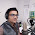## Sunday, January 1, 2017

### [Enrich20170101SQT] Calculating Square Roots by Hand

Introduction
Happy New Year to our readers!  I wish this year will be a fruitful one for everybody.
Today, I will illustrate how to calculate square roots by hand, using  54 756  as an example.  It is similar to long division, but has some modifications.

Solution

Starting from the right, pair up the digits.

2×2 = 4  is the nearest perfect square to  5.  Subtract and bring down the next two digits, giving  147.

Double the digit  2  to get  4.  Think:  ? × 4?  gives  147  or nearest possible value.  We have 3×43 = 129.

Subtracting and bringing down the next two digits gives  1856.  Replicate the digit  4  on the left and double the digit  3,  giving  46.

Now think:  ? × 46?  gives  1856  or nearest possible value.  It turns out that  4 × 464 gives exactly  1856.  We are done!  The square root of  54 756  is  234.

How does it work?

This relies on the algebraic identity  (10a + b)² = 100a² + 20ab + b², the right-hand expression is equal to   100a² + (20a + b)b.  For example, at stage 4, we have  a = 23,  b = 4  and  (20a + b) = 464.
Did you learn something today?

#### 1 comment:

1.Wow, man i really appreciate your efforts of calculating square by hand. I think you should also share your information on Qanda and other information sharing platform as well.

Note: Only a member of this blog may post a comment.